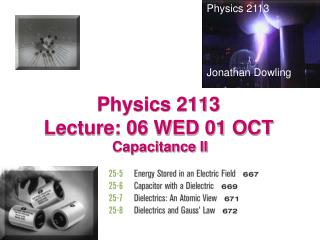DownloadDownload PresentationPhysics 2113 Lecture: 06 WED 01 OCT

# Physics 2113 Lecture: 06 WED 01 OCT

Télécharger la présentation## Physics 2113 Lecture: 06 WED 01 OCT

- - - - - - - - - - - - - - - - - - - - - - - - - - - E N D - - - - - - - - - - - - - - - - - - - - - - - - - - -
##### Presentation Transcript

1. Physics 2113 Jonathan Dowling Physics 2113 Lecture: 06 WED 01 OCT Capacitance II

2. V = VAB = VA –VB C1 Q1 VA VB A B C2 Q2 VC VD D C V = VCD = VC –VD Ceq Qtotal ΔV=V Capacitors in Parallel: V=Constant • An ISOLATED wire is an equipotential surface: V=Constant • Capacitors in parallel have SAME potential difference but NOT ALWAYS same charge! • VAB = VCD = V • Qtotal = Q1 + Q2 • CeqV = C1V + C2V • Ceq = C1 + C2 • Equivalent parallel capacitance = sum of capacitances PAR-V (Parallel: V the Same)

3. Q1 Q2 B C A C1 C2 Capacitors in Series: Q=Constant Isolated Wire: Q=Q1=Q2=Constant • Q1 = Q2 = Q = Constant • VAC = VAB + VBC SERI-Q: Series Q the Same Q = Q1 = Q2 • SERIES: • Q is same for all capacitors • Total potential difference = sum of V Ceq

4. C1 Q1 C2 Q2 Qeq Q1 Q2 Ceq C1 C2 Capacitors in Parallel and in Series • In parallel : • Cpar = C1 + C2 • Vpar= V1 = V2 • Qpar= Q1 + Q2 • In series : 1/Cser = 1/C1 + 1/C2 Vser= V1  + V2 Qser= Q1 = Q2

5. Parallel: Circuit Splits Cleanly in Two (Constant V) C1=10 μF C2=20 μF C3=30 μF 120V Example: Parallel or Series? • Qi = CiV • V = 120V on ALL Capacitors (PAR-V) • Q1 = (10 μF)(120V) = 1200 μC • Q2 = (20 μF)(120V) = 2400 μC • Q3 = (30 μF)(120V) = 3600 μC What is the charge on each capacitor? • Note that: • Total charge (7200 μC) is shared between the 3 capacitors in the ratio C1:C2:C3— i.e. 1:2:3

6. Series: Isolated Islands (Constant Q) Example: Parallel or Series C2=20mF C3=30mF C1=10mF What is the potential difference across each capacitor? • Q = CserV • Q is same for all capacitors (SERI-Q) • Combined Cser is given by: 120V • Ceq = 5.46 μF (solve above equation) • Q = CeqV = (5.46 μF)(120V) = 655 μC • V1= Q/C1 = (655 μC)/(10 μF) = 65.5 V • V2= Q/C2 = (655 μC)/(20 μF) = 32.75 V • V3= Q/C3 = (655 μC)/(30 μF) = 21.8 V Note: 120V is shared in the ratio of INVERSE capacitances i.e. (1):(1/2):(1/3) (largest C gets smallest V)

7. 10 μF 10μF 10μF 10V 5μF 5μF 10V Example: Series or Parallel? Neither: Circuit Compilation Needed! In the circuit shown, what is the charge on the 10μF capacitor? • The two 5μF capacitors are in parallel • Replace by 10μF • Then, we have two 10μF capacitors in series • So, there is 5V across the 10 μF capacitor of interest by symmetry • Hence, Q = (10μF )(5V) = 50μC

8. Energy U Stored in a Capacitor • Start out with uncharged capacitor • Transfer small amount of charge dq from one plate to the other until charge on each plate has magnitude Q • How much work was needed? dq

9. General expression for any region with vacuum (or air) volume = Ad Energy Stored in Electric Field of Capacitor • Energy stored in capacitor: U = Q2/(2C) = CV2/2 • View the energy as stored in ELECTRIC FIELD • For example, parallel plate capacitor: Energy DENSITY = energy/volume = u =

10. DIELECTRIC +Q –Q Dielectric Constant • If the space between capacitor plates is filled by a dielectric, the capacitance INCREASES by a factor κ • This is a useful, working definition for dielectric constant. • Typical values of κ are 10–200 C = κε0 A/d The κ and the constant ε=κεo are both called dielectric constants. The κ has no units (dimensionless).

11. Atomic View Emol Molecules set up counter E field Emol that somewhat cancels out capacitor field Ecap. This avoids sparking (dielectric breakdown) by keeping field inside dielectric small. Hence the bigger the dielectric constant the more charge you can store on the capacitor. Ecap

12. dielectric slab Example • Capacitor has charge Q, voltage V • Battery remains connected while dielectric slab is inserted. • Do the following increase, decrease or stay the same: • Potential difference? • Capacitance? • Charge? • Electric field?

13. dielectric slab Example • Initial values: capacitance = C; charge = Q; potential difference = V; electric field = E; • Battery remains connected • V is FIXED; Vnew = V (same) • Cnew =κC(increases) • Qnew = (κC)V = κQ(increases). • Since Vnew = V, Enew = V/d=E (same) Energy stored?u=ε0E2/2 => u=κe0E2/2 = εE2/2

14. Summary • Any two charged conductors form a capacitor. • Capacitance : C= Q/V • Simple Capacitors:Parallel plates: C = ε0 A/d Spherical : C = 4πε0ab/(b-a)Cylindrical: C = 2πε0L/ln(b/a) • Capacitors in series: same charge, not necessarily equal potential; equivalent capacitance 1/Ceq=1/C1+1/C2+… • Capacitors in parallel: same potential; not necessarily same charge; equivalent capacitance Ceq=C1+C2+… • Energy in a capacitor: U=Q2/2C=CV2/2; energy density u=ε0E2/2 • Capacitor with a dielectric: capacitance increases C’=κC